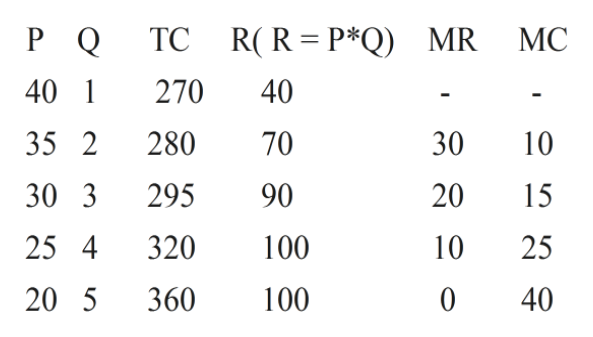# The above table provides some data for a monopoly firm. What is the profit maximizing point of production for this firm? Assume that the firm can only produce and sell whole units (discrete case).PriceQuantity DemandedTotal Cost\$401\$270352280303295254320205360A. 1B. 2C. 3D. 4E. 5

Question
53 views

The above table provides some data for a monopoly firm. What is the profit maximizing point of production for this firm? Assume that the firm can only produce and sell whole units (discrete case).

 Price Quantity Demanded Total Cost \$40 1 \$270 35 2 280 30 3 295 25 4 320 20 5 360

A. 1

B. 2

C. 3

D. 4

E. 5

check_circle

Step 1

Answer - The profit maximizing condition for monopolies are where marginal revenue (MR) is equal to marginal cost (MC) . If monopoly produces a lower quantity , than MR> MC at those levels of output, the firm can make higher profits by expanding output . If firm produces at a greater quantity, MC >MR , and the firm can make higher profits by reducing its quantity of output.
Now, from the table-help_outlineImage TranscriptioncloseP Q TC R(R=P*Q) MR MC 40 1 270 40 35 2 280 70 30 10 30 3 295 90 20 15 100 25 4 320 25 10 360 100 20 5 40 fullscreen
Step 2

From the table there is no pint where MR = MC . In that case Firm will produce upto the point where...

### Want to see the full answer?

See Solution

#### Want to see this answer and more?

Solutions are written by subject experts who are available 24/7. Questions are typically answered within 1 hour.*

See Solution
*Response times may vary by subject and question.
Tagged in